# Calibrate Radio Gain for Signal Capture

This example shows how to configure the front-end radio gain of your software-defined radio (SDR) hardware for your local environment. The example configures the SDR as a baseband transceiver to transmit a test waveform and to capture data from the air. The example then calculates and analyzes the average received signal power of the captured signals using different gain values.

### Introduction

Correctly setting the front-end radio gain plays an important role when you use your radio to capture radio frequency (RF) data from the air. Too much gain can lead to saturation that results in signal distortion. Too little gain hinders signal recovery. In this example, you use the `basebandTransceiver` object to calibrate the gain of the radio with a known transmit signal. For over-the-air signals, you can apply the same process using the `basebandReceiver` object.

The main steps of the gain calibration process are:

1. Configure a `basebandTransceiver` object to transmit a test waveform and then capture data at the correct sampling rate and center frequency (alternatively, you can configure a `basebandReceiver` object to capture data from the air).

2. Set the radio gain to the minimum gain value.

3. Capture the data, plot the time-domain waveform, and then calculate the average signal power and peak amplitude.

4. In the time-domain waveform, inspect how close the peak signal values come to the full-scale amplitude.

Call the `radioConfigurations` function. The function returns all available radio setup configurations that you saved using the Radio Setup wizard. For more information, see Connect and Set Up NI USRP Radios.

`savedRadioConfigurations = radioConfigurations;`

```savedRadioConfigurationNames = [string({savedRadioConfigurations.Name})]; radio =savedRadioConfigurationNames(1)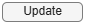;```

### Configure Baseband Transceiver

Create a baseband transceiver object with the specified radio. Because the object requires exclusive access to radio hardware resources, before running this example for the first time, clear any other object associated with the specified radio. In subsequent runs, to speed up the execution time of the example, reuse your new workspace object.

```if ~exist("bbtrx","var") bbtrx = basebandTransceiver(radio); end```

To update the dropdown menus with the antennas available for your radio, call the `hTransmitAntennas` and `hCaptureAntennas` helper functions. Then select the antennas to use with this example.

```transmitAntennaSelection = hTransmitAntennas(radio); captureAntennaSelection = hCaptureAntennas(radio); bbtrx.TransmitAntennas =transmitAntennaSelection(1); bbtrx.CaptureAntennas =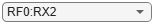captureAntennaSelection(1);```

Create a test waveform by using the `50ms_lte_data.bb` file. This baseband file contains 50 ms of LTE data captured from the air at a 30.72 MHz sampling rate.

```bbfr = comm.BasebandFileReader('50ms_lte_data.bb'); waveformInfo = info(bbfr); bbfr.SamplesPerFrame = waveformInfo.NumSamplesInData; transmitData = bbfr(); plotData(transmitData);```Set the transmit and capture sample rate and center frequencies. Set the capture duration to a value that enables the capture of enough data to show signal power variations. If you are working with a bursty signal, set the capture duration long enough to capture a burst. For details on how to work with bursty signals, see the Calibrate Radio Gain for Bursty Data section of this example.

```bbtrx.SampleRate = bbfr.SampleRate; bbtrx.CaptureCenterFrequency =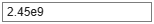2.45e9; bbtrx.TransmitCenterFrequency = bbtrx.CaptureCenterFrequency;```

Set the transmit radio gain and transmit the test waveform.

```bbtrx.TransmitRadioGain = 30; transmit(bbtrx,transmitData,'continuous');```

Because the test waveform in this example does not experience significant channel effects and the transmission is continuous, a capture duration of 200 ms is sufficient. When working with signals from the air, set the capture duration to a value that enables the capture of enough data to show signal power variations. If the signal is bursty, aim at capturing a complete burst.

`captureDuration = milliseconds(200);`

Set the radio gain to a value that provides a balance between sufficient received signal power for your application and sufficient headroom to avoid saturation from signal amplitude changes. To avoid damaging the receiver, set the capture radio gain to an initial value of 0 dB, and then capture data.

```bbtrx.CaptureRadioGain =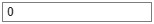0; data = capture(bbtrx,captureDuration);```

Plot the captured signal and calculate the average signal power relative to the full scale of the 16-bit integer range [-32768, 32767]. In the plot, the dotted red line represents the full-scale levels.

`plotData(data);`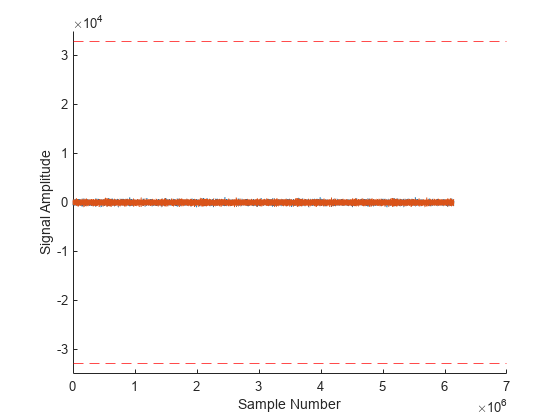```[pow,peak] = calcPowerAndPeak(data); disp("Average signal power is " + pow + " dBFS, peak amplitude is " + peak + ".");```
```Average signal power is -45.7666 dBFS, peak amplitude is 1098. ```

Adjust the gain to a value that meets your requirement for signal power and headroom. Increase the gain to 30 dB, plot the captured data, and calculate the average signal power. The captured data now occupies more of the dynamic range of the receiver than before while there is still some headroom for signal amplitude changes.

```bbtrx.CaptureRadioGain =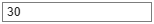30; data = capture(bbtrx,captureDuration); plotData(data);```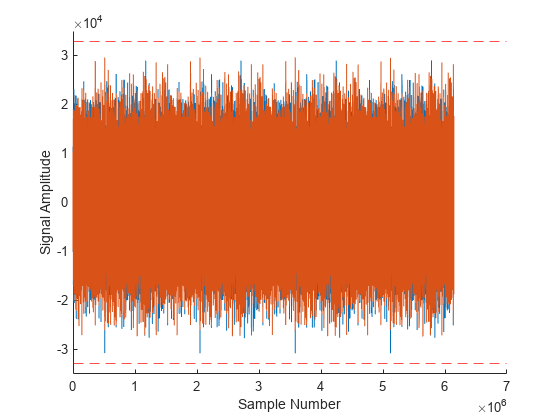```[pow,peak] = calcPowerAndPeak(data); disp("Average signal power is " + pow + " dBFS, peak amplitude is " + peak + ".");```
```Average signal power is -16.0269 dBFS, peak amplitude is 30776. ```

### Calibrate Radio Gain for Bursty Data

When you calibrate against a bursty signal, the average power in the signal capture does not represent the power of the signal of interest. To find the power of the signal of interest, you must isolate the signal of interest before calculating the power. Load a previously captured bursty WLAN signal and plot the data.

```load("200ms_ch60_wlan_data.mat"); plotData(data);``````[pow,peak] = calcPowerAndPeak(data); disp("Average signal power is " + pow + " dBFS, peak amplitude is " + peak + ".");```
```Average signal power is -43.3097 dBFS, peak amplitude is 5428. ```

To calculate the power in the signal of interest, use the Zoom In and Data Tips tools of the plot to obtain the sample number for the start and end of the signal of interest.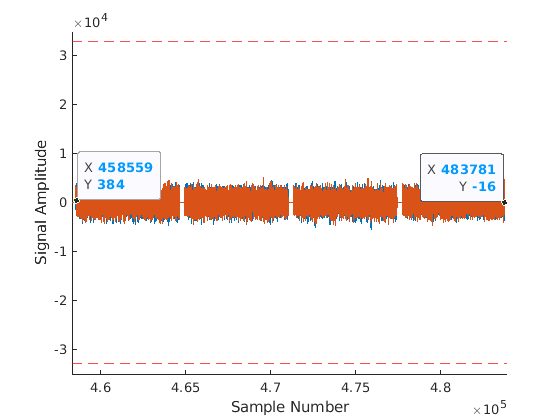Specify the sample numbers for the start and the end of the signal of interest using the values that are highlighted in the zoomed-in plot. Then calculate the average power and peak amplitude of the signal of interest.

```signalStartSample =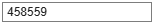458559; signalEndSample =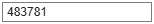483781; plotData(data(signalStartSample:signalEndSample));```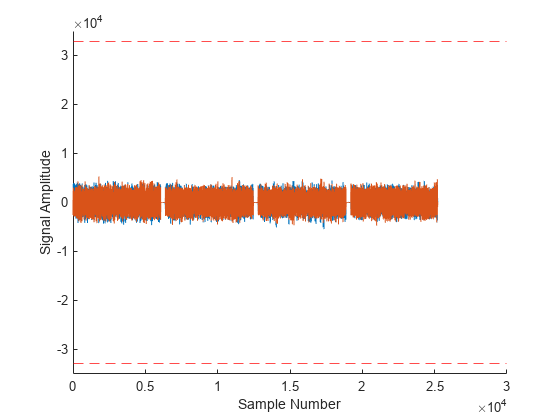```[pow,peak] = calcPowerAndPeak(data(signalStartSample:signalEndSample)); disp("Average signal power in the signal of interest is " + pow + " dBFS, peak amplitude is " + peak + ".");```
```Average signal power in the signal of interest is -24.3584 dBFS, peak amplitude is 5428. ```

Because the average power of the signal of interest is –24 dBFS, you can apply extra gain. In this case, you can increase the radio gain by 10 dB to 15 dB.

### Further Exploration

This example uses the `basebandTransceiver` object to show the calibration process with a known transmit signal. However, you can apply the same process to calibrate your radio for any signal from the air by using the `basebandReceiver` object. Once you calibrate the radio gain, you can use the gain value when you configure the radio gain for any Wireless Testbench™ application object. For an overview of available application objects, see Wireless Testbench Applications on SDR.

### Local Functions

```function plotData(data) figure(); hold on; ylim([-35000 35000]); plot(real(double(data))); plot(imag(double(data))); yline(intmin("int16"),"r--"); yline(intmax("int16"),"r--"); ylabel("Signal Amplitude"); xlabel("Sample Number"); end function [pow,peak] = calcPowerAndPeak(data) pow = pow2db(bandpower(double(data)./double(intmax("int16")))); peak = max(max(abs(real(data))),max(abs(imag(data)))); end```